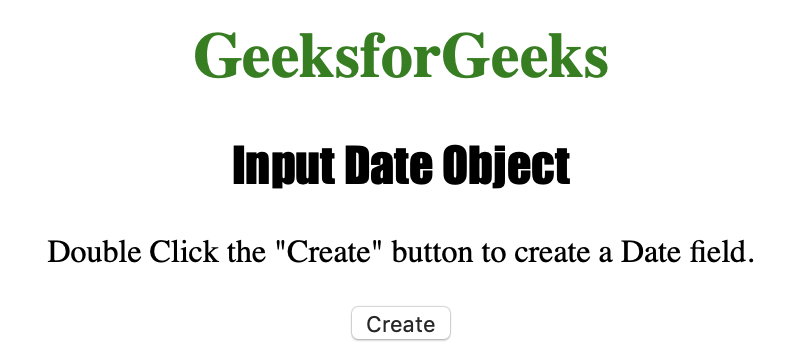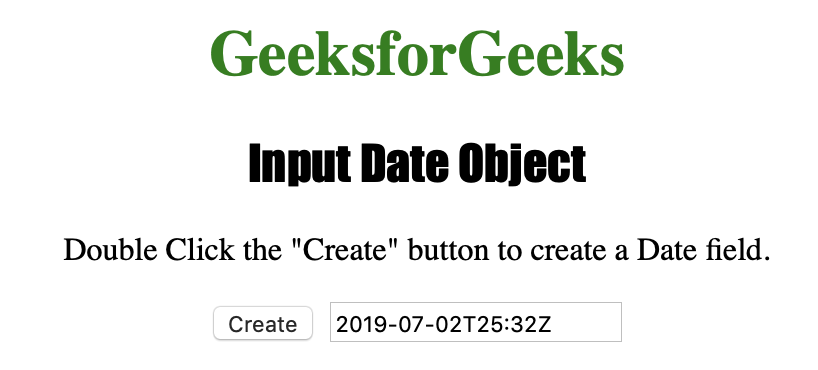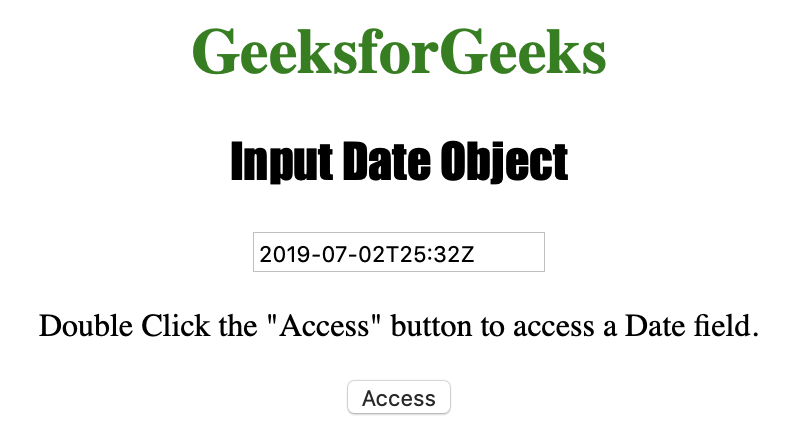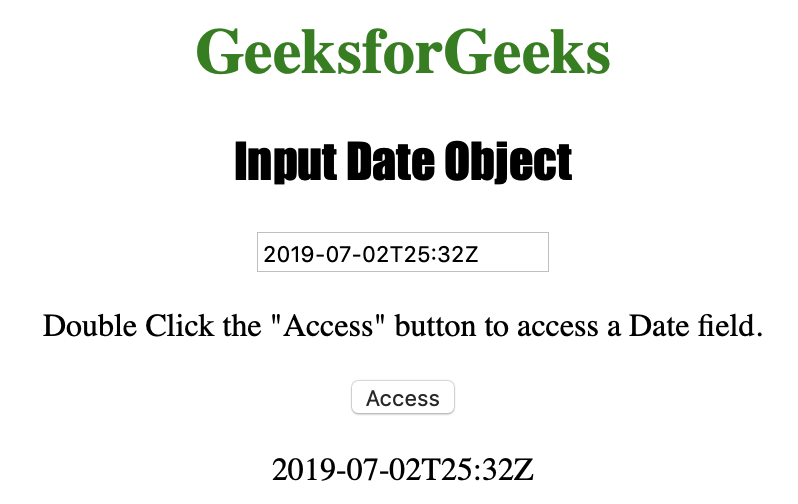# HTML | DOM Input Date Object

The Input Date object is used for representing an HTML <input> element of the type=”date”. The Input Date Object is a new object in HTML5.

Syntax:

• For creating a <input> element with the type =”date”:
```var gfg = document.createElement("input")
gfg.setAttribute("type", "date");```
• For accessing a <input> element with the type =”date”:
`document.getElementById("date_object")`

Property Values:

Input Date Object Methods:

• stepDown() : It is used for decrementing the value of the input date field by a specified number.
• stepUp() : It is used for incrementing the value of the input date field by a specified number.
• select() : It is used to select the content of the Input date field.

Below programs illustrate the Date Object:

Example-1: Creating a <input> element with the type =”date”.

## HTML

 `` `<``html``>`   `<``head``>` `    ``<``title``>Input Date Object` `    ``<``style``>` `        ``h1 {` `            ``color: green;` `        ``}` `        `  `        ``h2 {` `            ``font-family: Impact;` `        ``}` `        `  `        ``body {` `            ``text-align: center;` `        ``}` `    ``` ``   `<``body``>` `    ``<``h1``>GeeksforGeeks` `    ``<``h2``>Input Date Object` `    ``<``p``>Double Click the "Create" button ` `          ``to create a Date field.` `      ``` `    ``<``button` `ondblclick="Create()">Create` `      ```   `    ``<``script``>` `        ``function Create() {` `          `  `         ``// create input element type date.` `         ``var c = document.createElement("INPUT");` `         ``c.setAttribute("type", "date");` `         ``c.setAttribute("value", "2019-07-02T25:32Z");` `         ``document.body.appendChild(c);` `        ``}` `    ``` ``   ``

Output:

Before clicking the button:After clicking the button:Example-2: Accessing a <input> element with the type =”date”.

## HTML

 `` `<``html``>`   `<``head``>` `    ``<``title``>Input Date Object` `    ``<``style``>` `        ``h1 {` `            ``color: green;` `        ``}` `        `  `        ``h2 {` `            ``font-family: Impact;` `        ``}` `        `  `        ``body {` `            ``text-align: center;` `        ``}` `    ``` ``   `<``body``>` `    ``<``h1``>GeeksforGeeks` `    ``<``h2``>Input Date Object` `    ``<``input` `type="date" ` `           ``id="test" ` `           ``value="2019-07-02T25:32Z">` `    ``<``p``>Double Click the "Access" ` `          ``button to access a Date field.` `    ``<``button` `ondblclick="Access()">Access` `      ``` `    ``<``p` `id="check">`   `    ``<``script``>` `        ``function Access() {` `          `  `            ``// Accessing input element type =”date”` `            ``var a = document.getElementById(` `              ``"test").value;` `            ``document.getElementById(` `              ``"check").innerHTML = a;` `        ``}` `    ``` ``   ``

Output:

Before double clicking the button:After double clicking the button:Supported Browsers:

• Opera 11
• Firefox 57